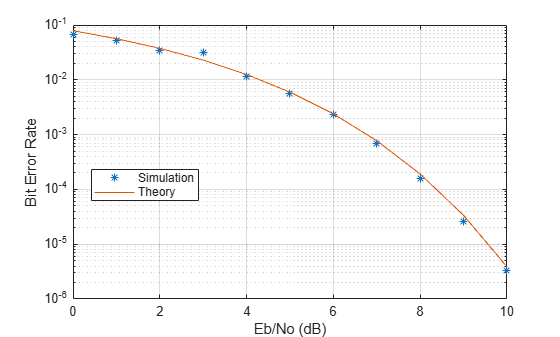Documentation

This is machine translation

Mouseover text to see original. Click the button below to return to the English version of the page.

QPSK and OFDM with MATLAB System Objects

This example shows how to simulate a basic communication system in which the signal is first QPSK modulated and then subjected to Orthogonal Frequency Division Multiplexing. The signal is then passed through an additive white Gaussian noise channel prior to being demultiplexed and demodulated. Lastly, the number of bit errors are calculated. The example showcases the use of MATLAB® System objects™.

Set the simulation parameters.

```M = 4; % Modulation alphabet k = log2(M); % Bits/symbol numSC = 128; % Number of OFDM subcarriers cpLen = 32; % OFDM cyclic prefix length maxBitErrors = 100; % Maximum number of bit errors maxNumBits = 1e7; % Maximum number of bits transmitted```

Construct System objects needed for the simulation: QPSK modulator, QPSK demodulator, OFDM modulator, OFDM demodulator, AWGN channel, and an error rate calculator. Use name-value pairs to set the object properties.

Set the QPSK modulator and demodulator so that they accept binary inputs.

```qpskMod = comm.QPSKModulator('BitInput',true); qpskDemod = comm.QPSKDemodulator('BitOutput',true);```

Set the OFDM modulator and demodulator pair according to the simulation parameters.

```ofdmMod = comm.OFDMModulator('FFTLength',numSC,'CyclicPrefixLength',cpLen); ofdmDemod = comm.OFDMDemodulator('FFTLength',numSC,'CyclicPrefixLength',cpLen);```

Set the `NoiseMethod` property of the AWGN channel object to `Variance` and define the `VarianceSource` property so that the noise power can be set from an input port.

```channel = comm.AWGNChannel('NoiseMethod','Variance', ... 'VarianceSource','Input port');```

Set the `ResetInputPort` property to `true` to enable the error rate calculator to be reset during the simulation.

`errorRate = comm.ErrorRate('ResetInputPort',true);`

Use the `info` function of the `ofdmMod` object to determine the input and output dimensions of the OFDM modulator.

`ofdmDims = info(ofdmMod)`
```ofdmDims = struct with fields: DataInputSize: [117 1] OutputSize: [160 1] ```

Determine the number of data subcarriers from the `ofdmDims` structure variable.

`numDC = ofdmDims.DataInputSize(1)`
```numDC = 117 ```

Determine the OFDM frame size (in bits) from the number of data subcarriers and the number of bits per symbol.

`frameSize = [k*numDC 1];`

Set the SNR vector based on the desired Eb/No range, the number of bits per symbol, and the ratio of the number of data subcarriers to the total number of subcarriers.

```EbNoVec = (0:10)'; snrVec = EbNoVec + 10*log10(k) + 10*log10(numDC/numSC);```

Initialize the BER and error statistics arrays.

```berVec = zeros(length(EbNoVec),3); errorStats = zeros(1,3);```

Simulate the communication link over the range of Eb/No values. For each Eb/No value, the simulation runs until either `maxBitErrors` are recorded or the total number of transmitted bits exceeds `maxNumBits`.

```for m = 1:length(EbNoVec) snr = snrVec(m); while errorStats(2) <= maxBitErrors && errorStats(3) <= maxNumBits dataIn = randi([0,1],frameSize); % Generate binary data qpskTx = qpskMod(dataIn); % Apply QPSK modulation txSig = ofdmMod(qpskTx); % Apply OFDM modulation powerDB = 10*log10(var(txSig)); % Calculate Tx signal power noiseVar = 10.^(0.1*(powerDB-snr)); % Calculate the noise variance rxSig = channel(txSig,noiseVar); % Pass the signal through a noisy channel qpskRx = ofdmDemod(rxSig); % Apply OFDM demodulation dataOut = qpskDemod(qpskRx); % Apply QPSK demodulation errorStats = errorRate(dataIn,dataOut,0); % Collect error statistics end berVec(m,:) = errorStats; % Save BER data errorStats = errorRate(dataIn,dataOut,1); % Reset the error rate calculator end```

Use the `berawgn` function to determine the theoretical BER for a QPSK system.

`berTheory = berawgn(EbNoVec,'psk',M,'nondiff');`

Plot the theoretical and simulated data on the same graph to compare results.

```figure semilogy(EbNoVec,berVec(:,1),'*') hold on semilogy(EbNoVec,berTheory) legend('Simulation','Theory','Location','Best') xlabel('Eb/No (dB)') ylabel('Bit Error Rate') grid on hold off```Observe that there is good agreement between the simulated and theoretical data.